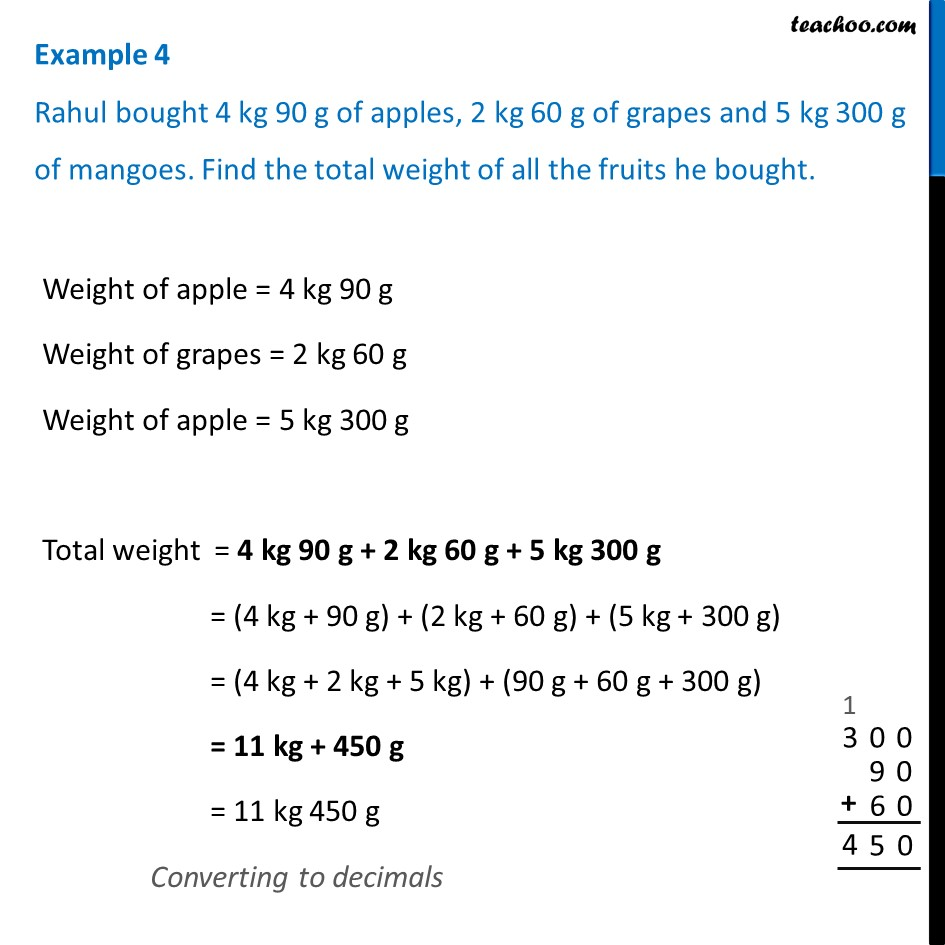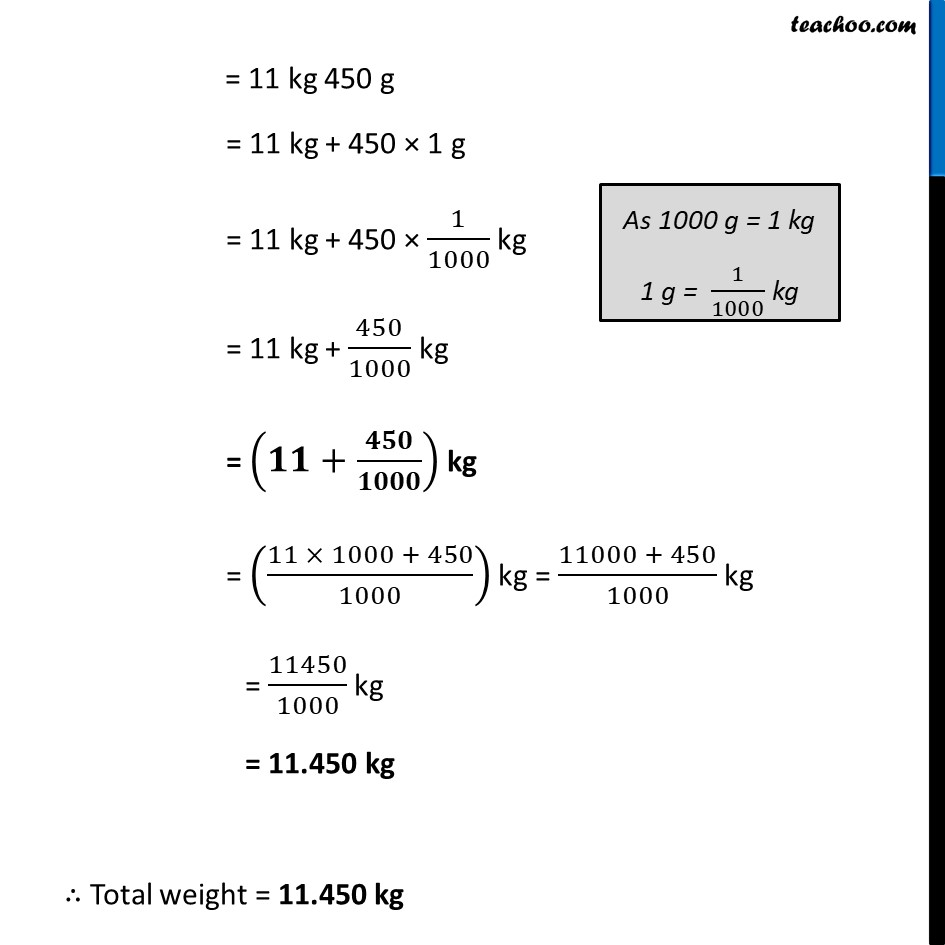Examples

Chapter 8 Class 6 Decimals
Serial order wiseLearn in your speed, with individual attention - Teachoo Maths 1-on-1 Class

### Transcript

Example 4 Rahul bought 4 kg 90 g of apples, 2 kg 60 g of grapes and 5 kg 300 g of mangoes. Find the total weight of all the fruits he bought.Weight of apple = 4 kg 90 g Weight of grapes = 2 kg 60 g Weight of apple = 5 kg 300 g Total weight = 4 kg 90 g + 2 kg 60 g + 5 kg 300 g = (4 kg + 90 g) + (2 kg + 60 g) + (5 kg + 300 g) = (4 kg + 2 kg + 5 kg) + (90 g + 60 g + 300 g) = 11 kg + 450 g = 11 kg 450 g Converting to decimals = 11 kg 450 g = 11 kg + 450 × 1 g = 11 kg + 450 × 1/1000 kg = 11 kg + 450/1000 kg = (𝟏𝟏+𝟒𝟓𝟎/𝟏𝟎𝟎𝟎) kg = ((11 × 1000 + 450)/1000) kg = (11000 + 450)/1000 kg = 11450/1000 kg = 11.450 kg ∴ Total weight = 11.450 kg